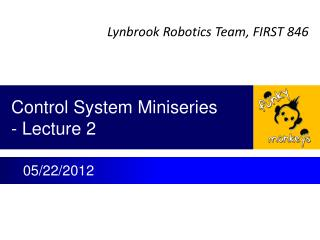# Control System Miniseries - Lecture 2 - PowerPoint PPT PresentationDownload PresentationControl System Miniseries - Lecture 2

Control System Miniseries - Lecture 2Download Presentation## Control System Miniseries - Lecture 2

- - - - - - - - - - - - - - - - - - - - - - - - - - - E N D - - - - - - - - - - - - - - - - - - - - - - - - - - -
##### Presentation Transcript

1. Control System Miniseries- Lecture 2 05/22/2012

2. Lecture 2 – What Will a Controller Do? • What we expect from a control system? • How to evaluate performance of a system • Typical performance requirements • Standard test approach • Typical system performance – demo • Control system design procedure

3. What do we expect from adding a controller to a system? Control Variable • With a control system, overall system should be • Stable • Quick response • Accurate • Resist disturbance Error + Controller Plant Input Output - Feedback Sensor System

4. Standard System Performance Tests Control Variable • One of standard system performance tests • Drive a system with step input • Observe output – system response Error + Controller Plant Input Output - Feedback Sensor System ?

5. Demo of Pendulum w/wo Damping • Make a pendulum • Get a bar with length ~ 2 – 3 feet long • Drill a hole at its top and insert rod (shaft) into the hole. • Note: The diameter of the rod should be much smaller than the hole diameter. • Hang the bar with the rod. Then, try to move top of pendulum a distance. You will see the pendulum oscillate less than 1 Hz. • You will find same length of bars, no matter of their cross section shape and weight, will have same oscillation frequency. • Make a damper • Find a container and fill with water • Dip lower end of pendulum into water. • Run the test • At different dipping depth, move top of pendulum a distance (step input) • Observe pendulum response. Step Move

6. Under-damping Pendulum Pendulum above water (no damping) Under-damping system

7. Optimal-damping Pendulum Dip pendulum into water to a proper depth optimal-damping system

8. Over-damping Pendulum Dip pendulum into water to deeper over-damping system

9. Pendulum with Short Length Step Move Lower oscillation frequency. Similar step response Step Move Higher oscillation frequency.

10. Goal of Control System Design • There are varieties of plants (devices, systems) • Mechanical/Pneumatic/Electrical/ Hybrid • These plants have their own characteristics • Different mathematical expression • But, after add proper controllers and control loop, overall systems should have same response to step input as optimal-damped pendulum • Pendulum with damping can be mathematically modeled as 2nd order differential equation. • Control system design will make any system have same mathematically expression (behavior) as the pendulum. • So, overall system will have quick and accurate response. Where ζ – damping ratio ωb(= 2πfb) – control system bandwidth

11. Control System Design Procedure • Define system spec • Stability, response time, accuracy, robustness, reliability, etc. • Analyze plant • Modeling based on physics and math • Design controller and control loop • Example PID controller • Modeling • Run simulation • Make system meet spec • Mathematically, overall system can be expressed as 2nd order differential equation with optimal damping ratio (ζ = 0.5 ~ 1, ωb = 5 - 10 Hz for 50 Hz system sample rate) • Experimentally, run step input response. P Plant Input Output I D Control Variable Input Error + Plant Output - Controller Feedback Sensor

12. Example Motor Output Torque Gearbox Output Torque Calculated Wheel Speed Speed Error Control Voltage Motor Voltage Wheel Speed Δω (rpm) Vctrl (volt) Vm (volt) Tm (N-m) Tgb (N-m) ωwhl (rpm) ω0 (rpm) + Control Software Jaguar Speed Controller Motor Gearbox Shooter Wheel - Controller Plant Sensor Voltage to Speed Converter Hall Effect Sensor (Voltage Pulse Generator Pulse Counter ωfbk (rpm) Vpls (volt) Pwhl (# of pulse) Measured Wheel Speed Voltage of Pulse Rate Sensor Pulse • Present every major component • Label variables and physical unit • Label conversion factor